# Calculating time in Google Sheets: subtract, sum and extract date and time units

Now, that we've learnt how to enter dates and time to your spreadsheet, it's time to talk about the ways of calculating time in Google Sheets. We'll discuss the ways of finding time difference in detail, see how to sum dates and time together, and learn to display only date or time units and set them apart completely.

## How to calculate time difference in Google Sheets

When you're working on some projects, it is usually important to control how much time you spend. This is called elapsed time. Google Sheets can help you calculate the time difference in a lot of various ways.

### Example 1. Subtract time to get the time duration in Google Sheets

If you have your start time and end time, it's not a problem to find out the time spent:

= End time - Start time

Let's assume the start time is in column A and the end time is in column B. With a simple subtraction formula in C2, you will find how much time this or that task took:

`=B2-A2`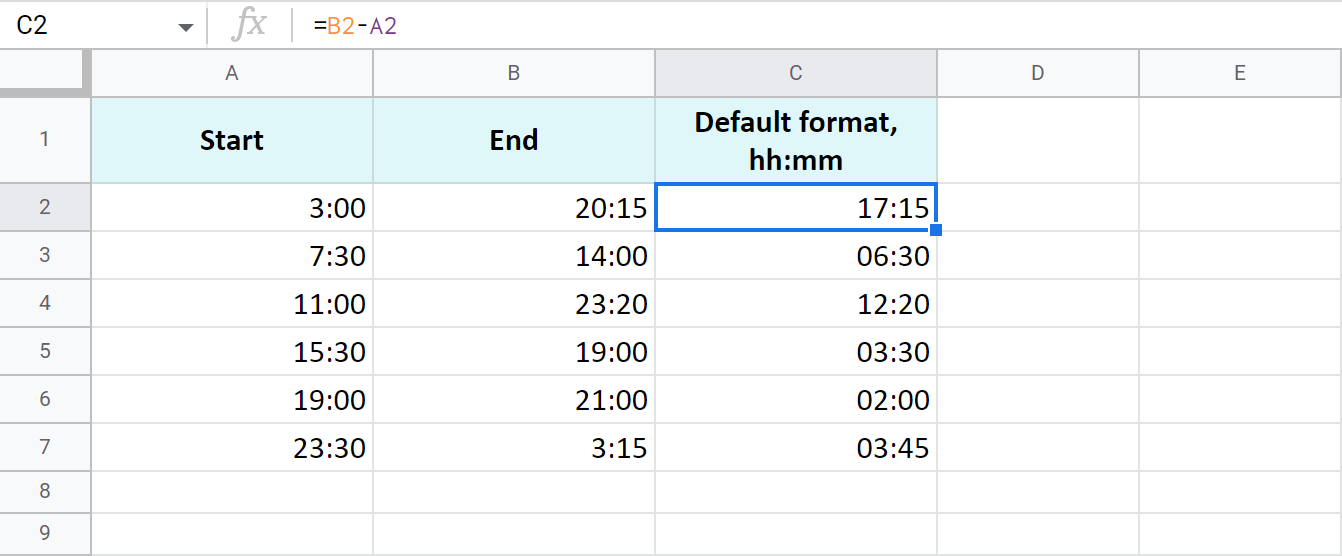The time is formatted as "hh:mm" by default.

To get the results as hours only or as hours, minutes, and seconds, you need to apply a custom format with the corresponding time codes: h and hh:mm:ss. Google even offers a special number format for cases like this - Duration: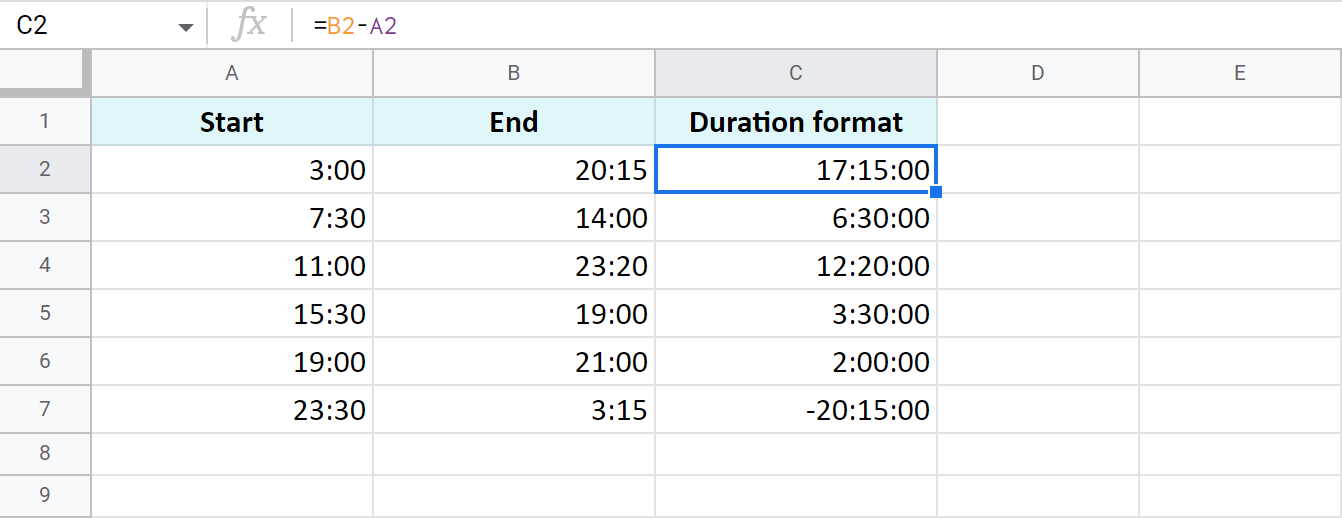Tip. To apply the custom time format, go to Format > Number > More Formats > Custom number format in your spreadsheet menu.

### Example 2. Calculate time duration in Google Sheets using the TEXT function

Another trick to calculate the time duration in Google Sheets involves the TEXT function:

`=TEXT(B2-A2,"h")` - for hours

`=TEXT(B2-A2,"h:mm")` - for hours and minutes

`=TEXT(B2-A2,"h:mm:ss")` - for hours, minutes, and seconds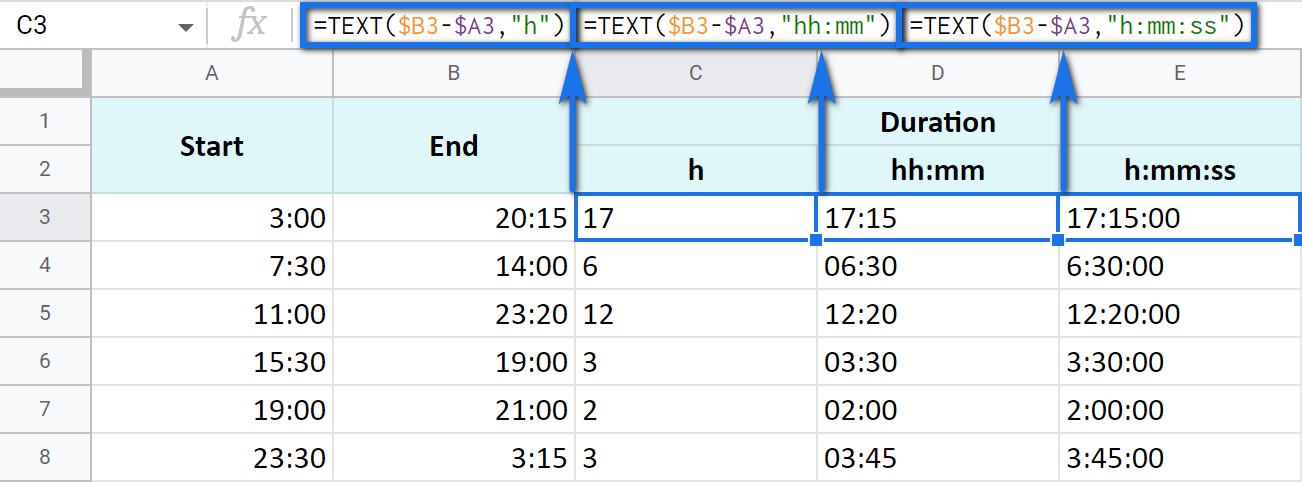Note. See how the records are aligned to the left? Because the TEXT function always returns the results formatted as a text. This means these values cannot be used for further calculations.

### Example 3. Time difference in hours, minutes, and seconds

You can track the time spent and get the result in one time unit disregarding other units. For example, count the number of only hours, only minutes, or only seconds.

Note. To ensure correct results, your cells should be formatted either as numbers or automatically: Format > Number > Number or Format > Number > Automatic.

• To get the number of hours spent, subtract your start time from the end time and multiply the result by 24 (since there are 24 hours in one day):

=(End time - Start time) * 24

You will get a time difference as a decimal: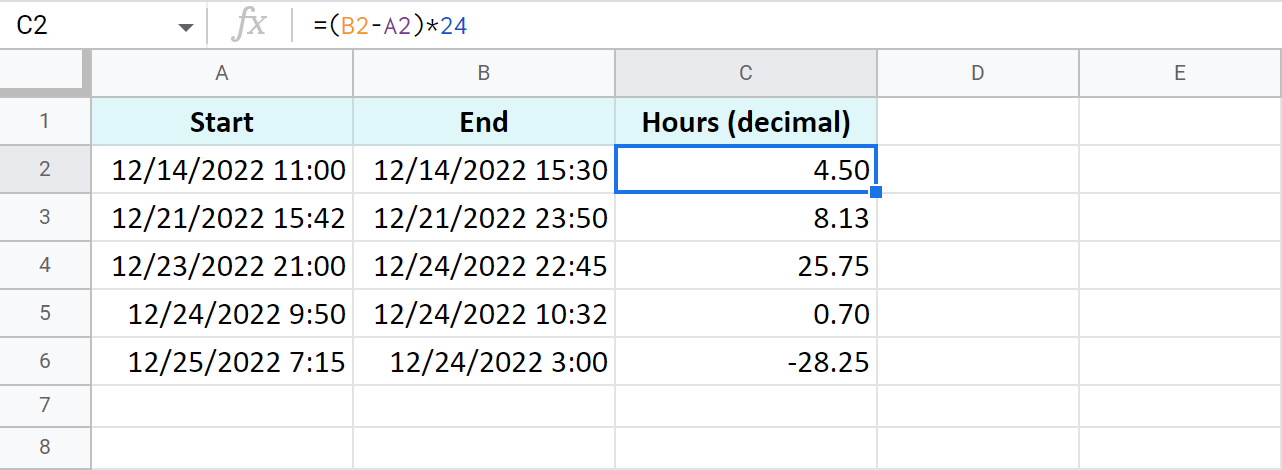If the start time is greater than the end time, the formula will return a negative number, like in C5 in my example.

Tip. The INT function will let you see the number of complete hours spent since it rounds numbers down to the nearest integer: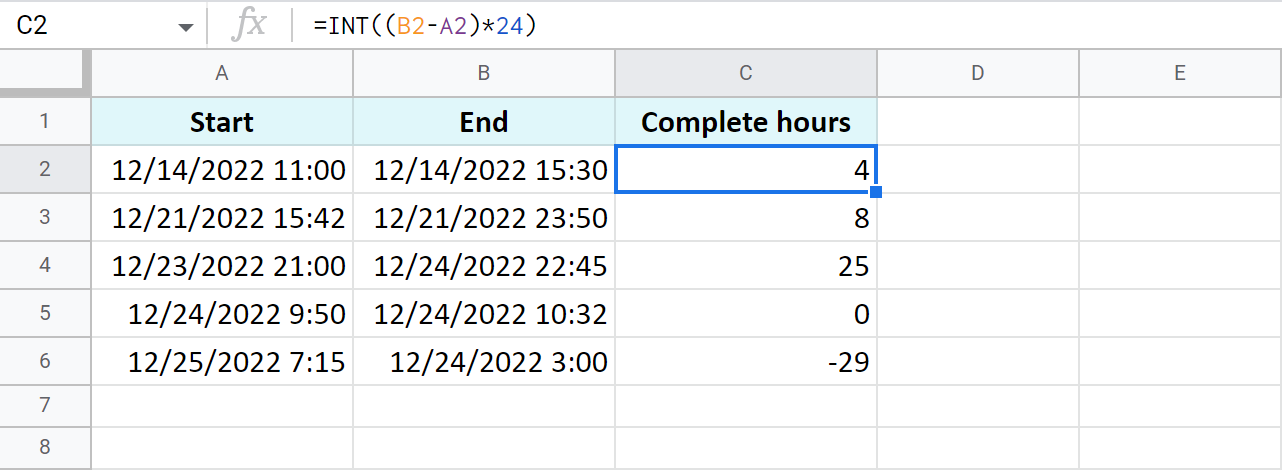• To count minutes, substitute the start time from the end time and multiply whatever you get by 1,440 (since there are 1,440 minutes in one day):

=(End time - Start time) * 1440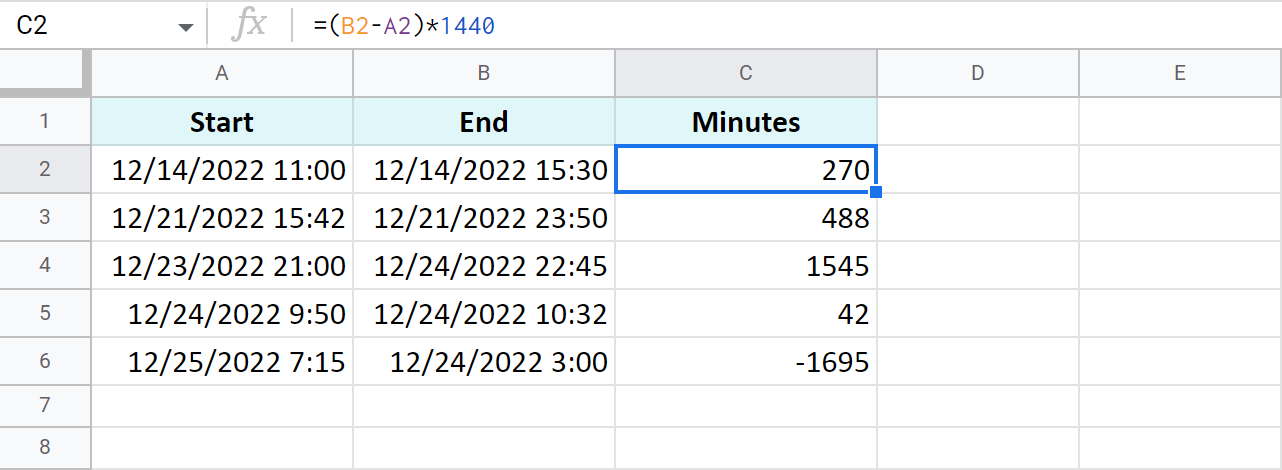• To find out how many seconds passed between two times, the drill is the same: substitute the start time from the end time and multiply the result by 86,400 (the number of seconds in a day):

=(End time - Start time) * 86400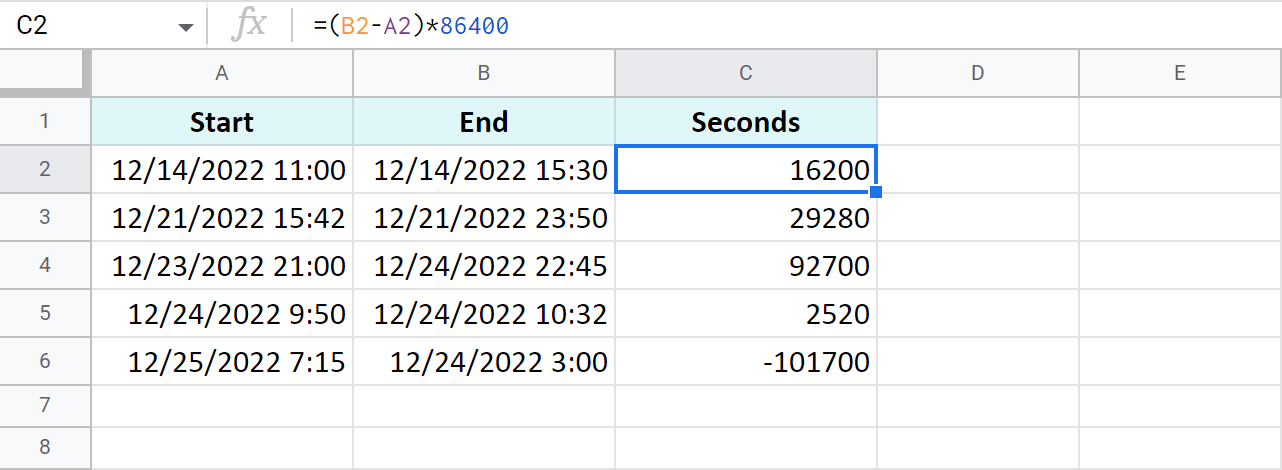Tip. You can avoid multiplying in all these cases. Just subtract times first, and then apply elapsed time format from Format > Number > More Formats > More date and time formats. If you click the down arrow to the right of the text field, you'll be able to choose between additional date and time units: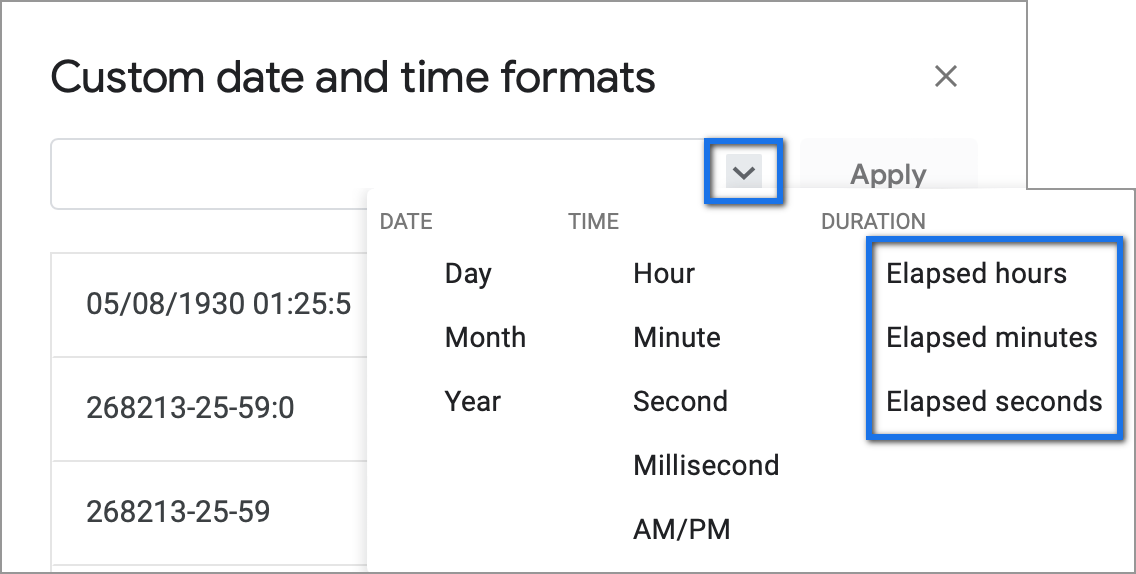### Example 4. Functions to get the time difference in a Google spreadsheet

As always, Google Sheets equips you with three particularly useful functions for this purpose.

Note. These functions work only within 24 hours and 60 minutes and seconds. If the time difference exceeds these limits, the formulas will return errors.

• `=HOUR(B2-A2)` - to return hours only (without minutes and seconds)
• `=MINUTE(B2-A2)` - to return minutes only (without hours and seconds)
• `=SECOND(B2-A2)` - to return seconds only (without hours and minutes)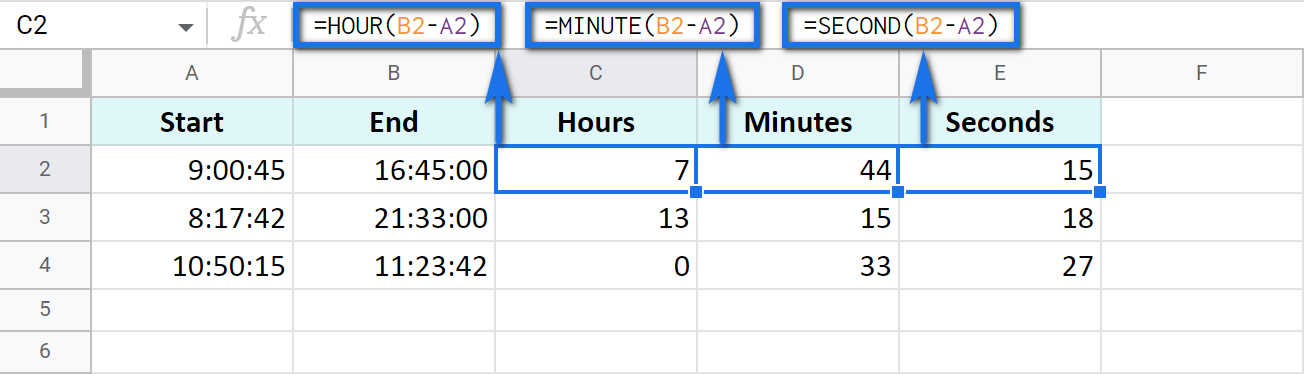## How to add and subtract time in Google Sheets: hours, minutes, or seconds

These operations can also be achieved with two techniques: one involves basic math calculations, another - functions. While the first way always works, the second one with functions works only when you add or subtract units less than 24 hours, or 60 minutes, or 60 seconds.

• Add less than 24 hours:

=Start time + TIME(N hours, 0, 0)

Here's how the formula looks on real data:

`=A2+TIME(3,0,0)`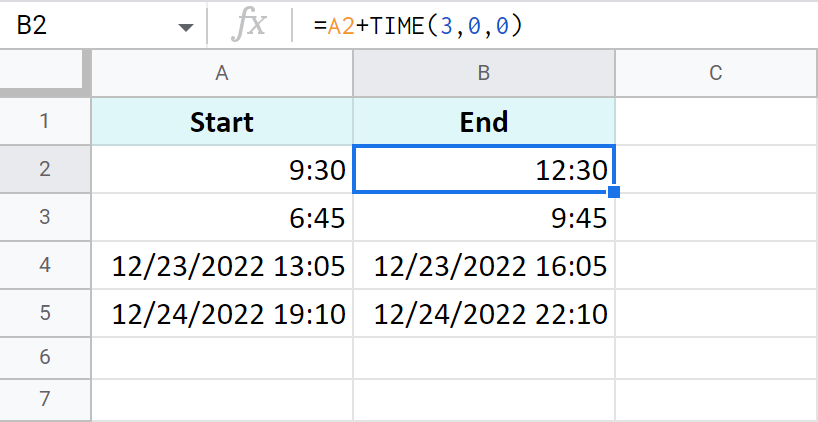• Add more than 24 hours:

=Start time + (N hours / 24)

To add 27 hours to the time in A2, I use this formula:

`=A2+(27/24)`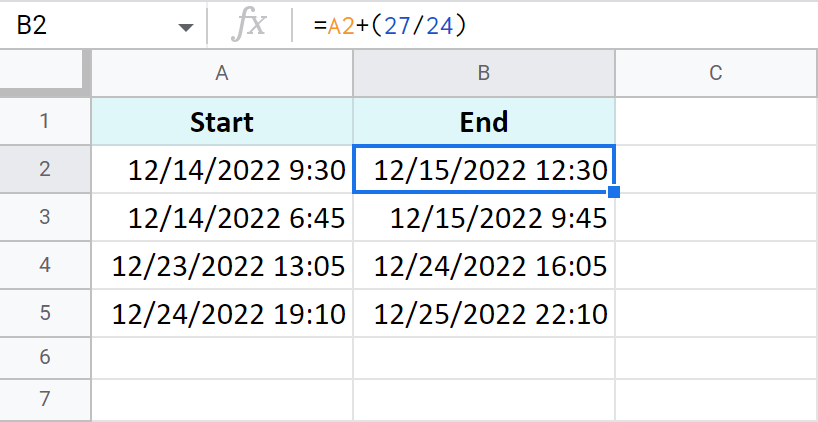• To subtract 24 and more hours, use the formulas above as a basis but change the plus sign (+) to the minus sign (-). Here's what I've got:

`=A2-TIME(3,0,0)` - to subtract 3 hours

`=A2-(27/24)` - to subtract 27 hours

The principle of manipulating minutes is the same as with the hours.

• There's the TIME function that adds and subtracts up to 60 minutes:

=Start time + TIME(0, N minutes, 0)

If you are to add 40 minutes, you can do it like this:

`=A2+TIME(0,40,0)`

If you are to subtract 20 minutes, here's the formula to use:

`=A2-TIME(0,40,0)`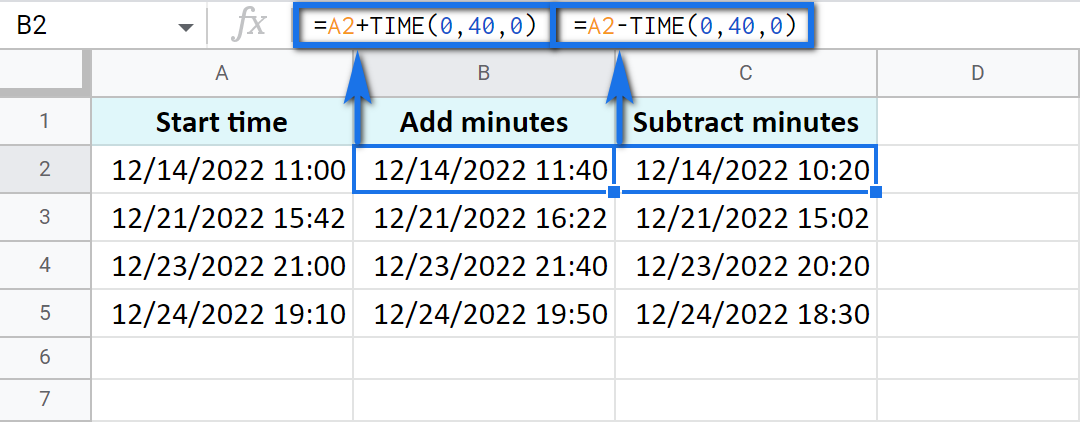• And there's a formula based on simple arithmetic to add and subtract over 60 minutes:

=Start time + (N minutes / 1440)

Thus, here's how you add 120 minutes:

`=A2+(120/1440)`

Put the minus instead of plus to subtract 120 minutes:

`=A2-(120/1440)`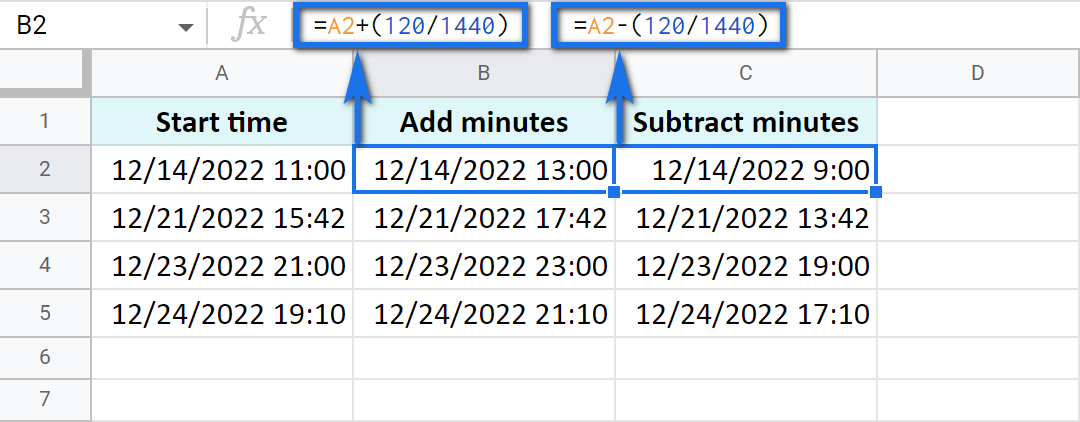Seconds in Google Sheets are calculated in the same manner as hours and minutes.

• You can use the TIME function to add or subtract up to 60 seconds:

=Start time + TIME(0, 0, N seconds)

`=A2+TIME(0,0,30)`

Or substitute 30 seconds:

`=A2-TIME(0,0,30)`

• To calculate over 60 seconds, use simple maths:

=Start time + (N seconds / 86400)

`=A2+(700/86400)`

Or substitute 700 seconds:

`=A2-(700/86400)`

## How to sum time in Google Sheets

To find the total time in your table in Google Sheets, you can use the SUM function. The trick here is to choose the correct format to display the result.

By default, the result will be formatted as Duration - hh:mm:ss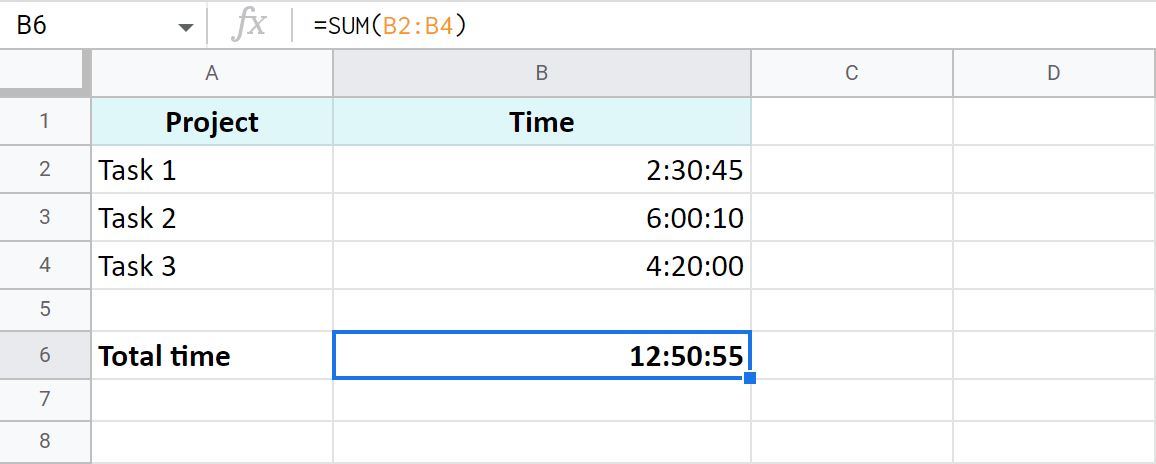But most often the default time or duration format won't be enough, and you will need to come up with your own one.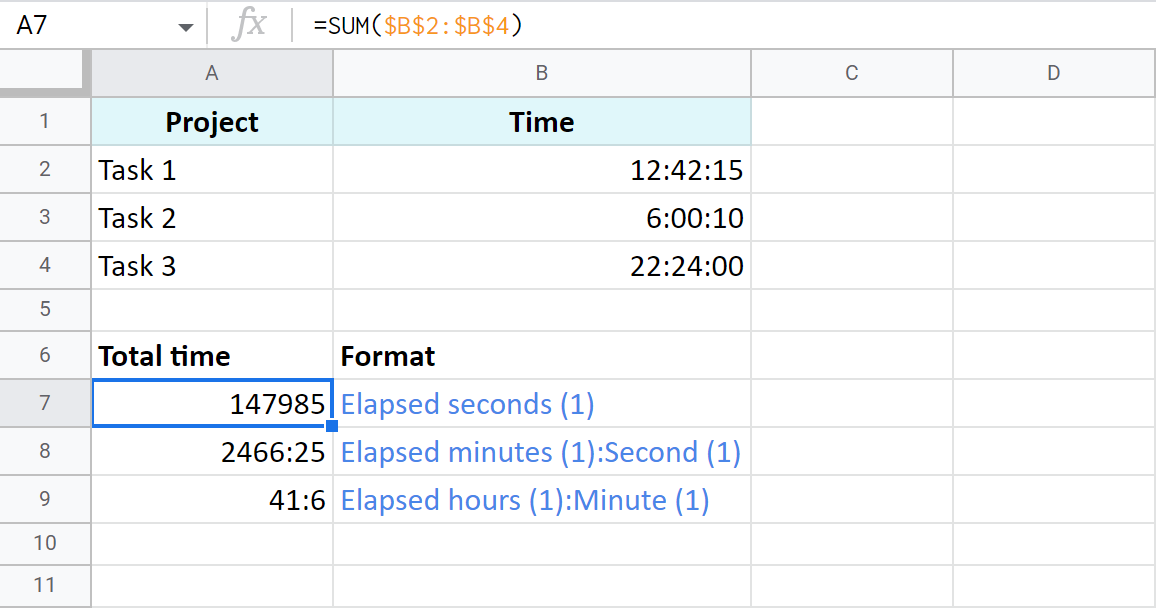A7:A9 cells contain the same time value. They are just displayed differently. And you can actually perform calculations with them: subtract, sum, convert to decimal, etc.

## Extract date and time from a full "date-time" record

Let's imagine that one cell in Google Sheets contains both, date and time. You want to set them apart: extract only the date to one cell and only time to another.

### Split Date time using Number format

In order to display date or time in one cell on your screen or to print it, just select the original cell, go to Format > Number and choose Date or Time.

However, if you'd like to use these values for future calculations (subtract, sum, etc.), this won't be enough. If you don't see the time unit in a cell, it doesn't necessarily mean that it's absent, and vice versa.

So what do you do?

### Split Date time using formulas

Google stores dates and time as numbers. For example, it sees the date 8/24/2017 11:40:03 as the number 42971,4861458. The integer part represents the date, the fractional - time. So, your task is down to separating integer from fractional.

1. To extract date (integer part), use the ROUNDDOWN function in cell B2:

`=ROUNDDOWN(A2,0)`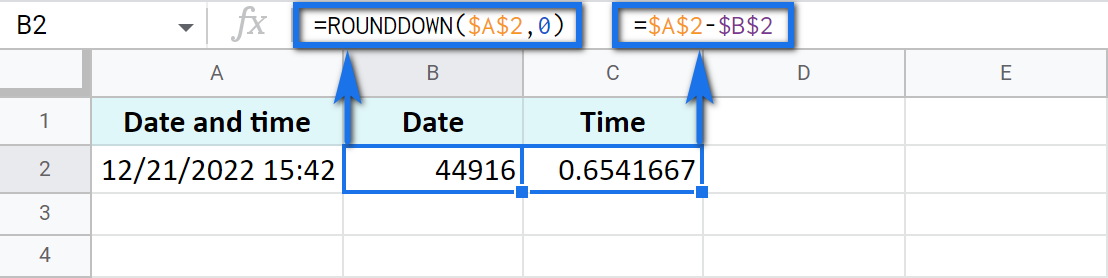The formula rounds the value down and casts the fractional part away.

2. To extract time, place the following subtraction formula into C2:

`=A2-B2`

3. Copy the results into the third row and apply Date format to B3 and Time format to C3: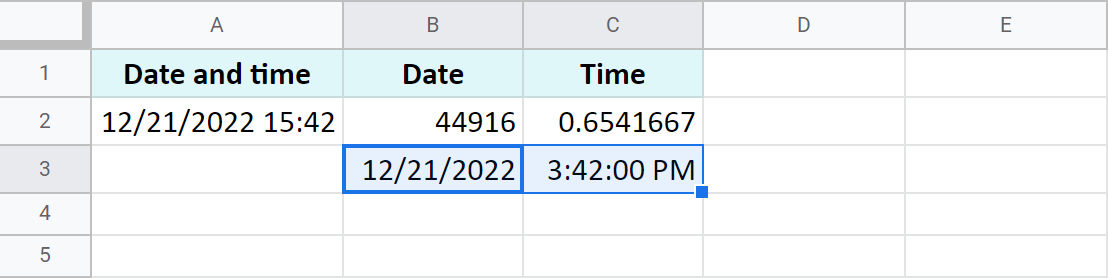### Use the Split Date & Time add-on

You may be surprised but there's one special add-on for this job. It's really small and easy but its contribution to Google Sheets cannot be overstated.

Split Date & Time splits all Date time records in your entire column at once. You control the desired outcome with just 4 simple settings: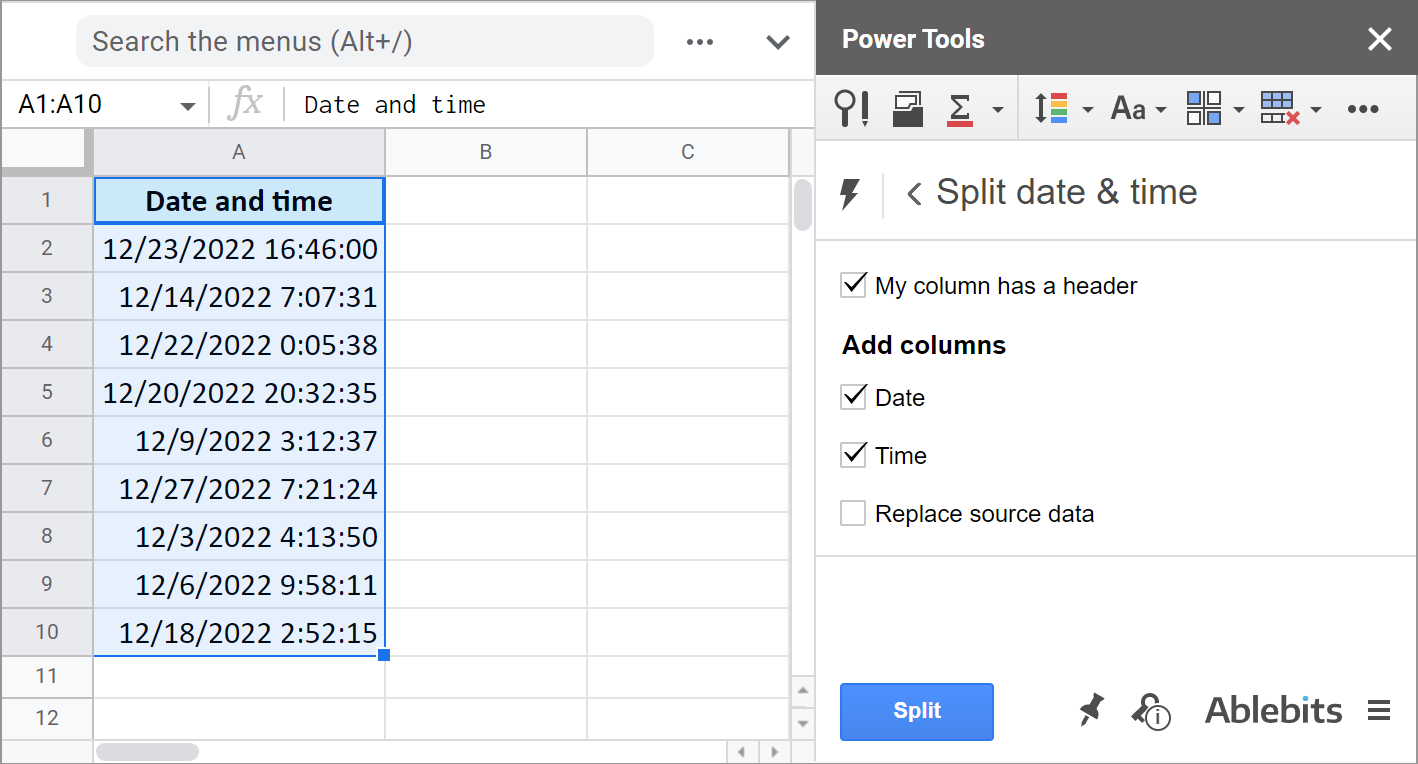1. Whether there's a header row.
2. If you want to get the Date unit.
3. If you want to get the Time unit.
4. And if you'd like to replace your original column with the new data.

It literally takes the burden of splitting date and time units off your shoulders: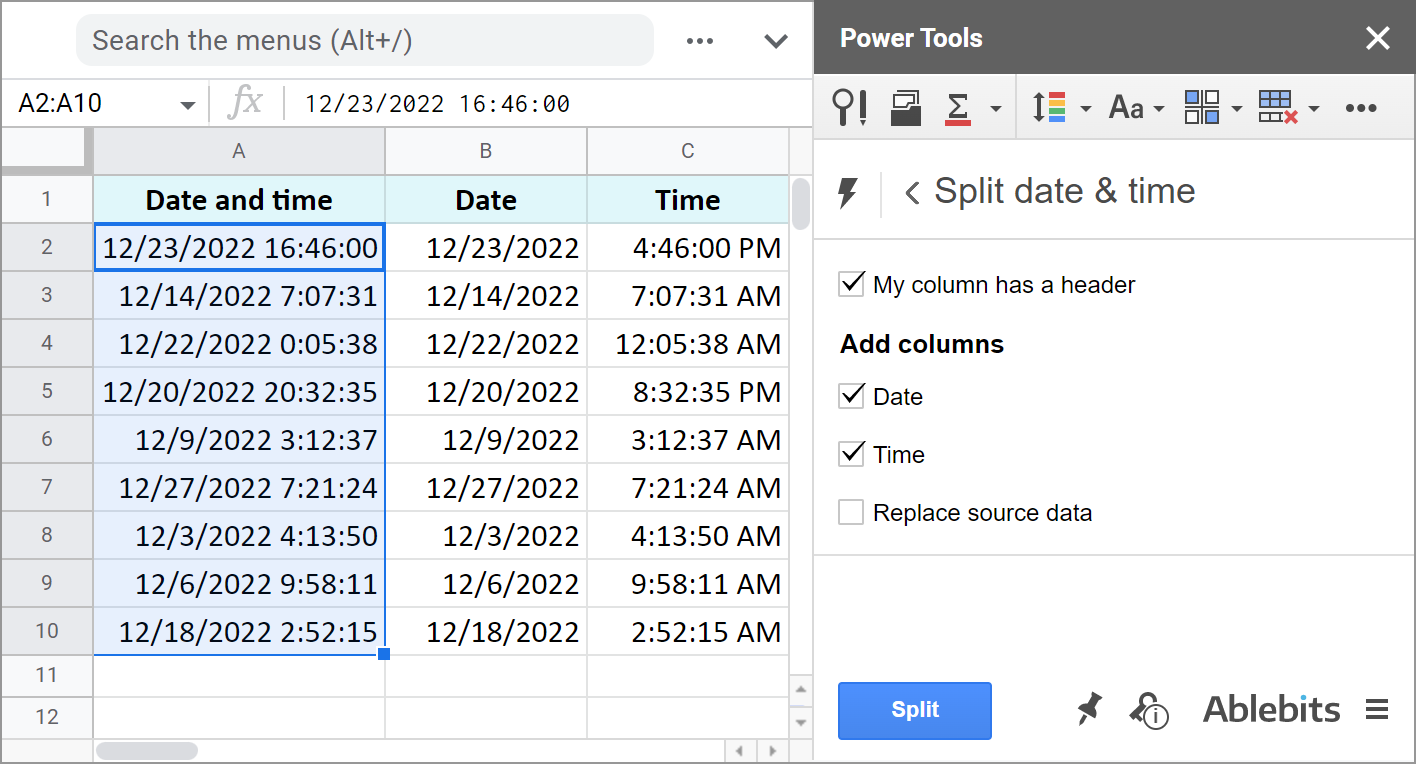The add-on is part of the Power Tools collection so you will have more than 30 other useful add-ons at hand. Install it from the Google Sheets store to test everything out.

These are the ways to not only display date or time, but to separate them to different cells. And you can perform various calculations with these records now.

## You may also be interested in

1. Somewhere in all this there may be a solution to my issue but I am not seeing it. I need to calculate total elapsed time between values in 2 cells which are in the format of MM/DD/YYYY HH:MM:SS, as it Start Date/Time and End Date/Time. It is not feasible to split this into 4 columns as the data is from a ticketing system and gives one value as the start and one as the end.

For example, the elapsed time between 08/22/2023 08:00:00 and 08/22/2023 09:00:00 is 0 days 1:00 hour. That calculation works fine. But, the elapsed time when it spans days AND the format in each cell is as noted eludes me. The elapsed time between 08/22/2023 08:00:00 and 08/23/2023 09:00:00 is 25 hours and I can't get it to do both in the same group of 2 columns, with the resulting elapsed time in DD:HH:MM:SS or some similar format, e.g. 1 day 1 hour 0 minutes 0 seconds, etc.

Suggestions?

• Hi David,

Sorry, could you please specify the formulas you use to calculate your elapsed times? And also specify the exact formats you're applying to your cells.

2. Hi Natalie,

I have a sign-in sheet that when you select your name from a drop down menu the computer returns the time you signed in. I want a formula that calculates how many minutes you sign in late beyond 7:30. Also I want any time signed in beyond 7:30 to turn red.

Many thanks.

• Hi Hayden,

To calculate minutes, you need to subtract 7:30 from the actual sign in time. You'll find formula examples here.

To color cells red as well, you need to use conditional formatting.

3. putting time data into specific time groups in google sheets
like 1:00,2:00,13:00,15:00,17:00 in group1 1pm-6Pm = 2, group2 13:00-17:00=3

is there any formula which can help

4. Hi

I am trying to use google sheets to work out the fast time across a number of rows. I have set the format of the time cells as custom: MM:SS.00

The formula I am trying to use is =MIN(D6:N6) - however the result is coming back as 00:00.00

I've tweaked the formula to exclude any empty cells, but its still coming back at 00:00.00 - any tips would be massively appreciated!

The time format is merely what you see in cells. Google Sheets still works with values behind the format.

You may also share an editable copy of your spreadsheet with me (support@apps4gs.com) so I could look directly into the file. I kindly ask you to shorten the table to 10-20 rows.
Note. We keep that Google account for file sharing only and don't monitor its Inbox. Please do not email there. Once you share the file, just confirm by replying to this comment.

5. Its not working in mobile app

• Hello Md Imran Akhter,
Could you please specify what doesn't work exactly?

• hi @Natalia i need to find the total number of time formats(00:00 - 23:59) updated in the sheet

• Hi Raj,

Sorry, what do you mean by "updated formats"? Do you need the number of cells with the non-standard time format? And which one you regard as the standard one?

6. How do I divide two durations? For example.... I have 1,216 hours of available time on my team in a given month. It takes the team 28 minutes to produce a widget. I want to know how many widgets they can produce in the 1,216 hours. The result should be in whole numbers not duration.

Basically I'm looking to calculate how many times 28 minutes goes into 1,216 hours. Thanks

• Hello Jon,

Assuming the hours are in A2, here's the formula:
=ROUND(A2*60/28,2)

1. A2*60 converts hours to minutes.
2. It's divided by 28 to find out the number of widgets.
3. ROUND rounds the result to 2 decimals.
7. what about the negative value ie. PLANT OUT ETA
10:52:00, 15:52:00 =?
07:18:00, 12:18:00 =?
00:46:00, 5:46:00 =?

• Hello vikas,

I'm sorry but your task is not clear. Please describe it in detail including the formulas you're trying to use. I'll do my best to help.

8. I am working on a competition schedule. Depending on the levels(there could be 30) there is a different time allotted for each competitor. IF(G4=1,"30 MINUTE LESSON" is an example of one of my time formulas. in the next column, I have if(H3="30 MINUTE LESSON","00:30" as an example in my time column. All of this is working fine. However, on my last column I am simply adding my 00:30 from the last formula I have shown to a time that I start at the top of the column. That is a manually entered time depending on when the competition begins. Everything is working, except when we try and move rows, this last column will not update the correct time. Help?

When scheduling competitors they need to be moved around to ensure they are at the right time and in the right group.

• Hello Crissteen,

For me to be able to help you, please share an editable copy of your sheet with me (support@apps4gs.com) and specify where the formulas that don't update correctly are. If you have confidential information there, you can replace it with some irrelevant data, just keep the format.

Note. We keep that Google account for file sharing only and don't monitor its Inbox. Please do not email there. Once you share the file, just confirm by replying to this comment.

I'll try to help.

9. I am facing a problem that want to write a function which will return date and time, example below

function setD(x) {
if(x != ""){
const d = new Date();
return d;
}
}

This function returning a value which correct date which value looks : 4/21/2023

I want to return my computer time which value should looks like : 04:35 AM

I'm not able to find that time function which I possible excecated in the function and return a time value only, can you help me ?

• Oh thanks a lot I got it. and if anybody need then you can take it from here :

function setT(y){
if(y != ""){
const a = new Date().getHours();
if( a < 12 ){
const c = 'AM';
const b = new Date().getMinutes();
const Its_Time = a + ':' + b + ' ' + c;
return Its_Time;
}else{
const d = a - 12;
const c = 'PM';
const b = new Date().getMinutes();
const Its_Time = d + ':' + b + ' ' + c;
return Its_Time;
}
}
}

10. Hi Natalia,

I am subtracting the hours accumulated for vacation time remaining. I'm trying to figure out how many days left in my vacation time.
If I have 72 vacation hours (H3) and have used 3 hours (H4), 3 hours (H5) and 24 hours (H6) and now have 42 hours (H11=H3-H4-H5-H6-H7-H9-H10) remaining, how do I calculate or how do I format cell H12 to reflect the remaining days left if I work 3.5 days/ week (7.5 hours each)?

Hope this is clear.

Thank you!

• Hi fabio,

=(H11*24)/7.5

Multiply H11 by 24 to return hours as a decimal first. Then divide it by the number of hours you work daily. I've got 5.60 days.

If this is not exactly what you need, please specify what the result should be and elaborate on the last part of your task: "reflect the remaining days left if I work 3.5 days/ week (7.5 hours each)"

11. Hi Natalia,

I am currently tracking my task durations in google sheets, which I then add up (or subtract) to calculate the total time duration in the same column.

I am currently using the format : Elapsed hours (1) h: Minute (1) m
The end result is as expected, for e.g : 0h:30m , 5h:0m etc

Is there a way to hide the hours (h) or minutes (m) if they are 0?
So from the example above, I'd like it to display as 30m and 5h only.
What would be the right format to use to achieve this?

• Hi Drake,

If you set up the format using the Google Sheets number format, it's going to show minutes/hours even if they're zero units.
To hide zero values, I believe you need to incorporate the IF function & check if the result has 0 minutes or 0 hours. Then return the result in the required format set up by the TEXT function in the formula itself.

12. Thanks for the post above on calculating times. I'm struggling to calculate negative times.

I have a spreadsheet that calculates the duration of a shift from the start and finish time.

What I'm trying to achieve is a formula that works out the difference,negative or positive, between a standard 8hr shift.

Any ideas?

Thanks

James

• Hello James,

Could you please describe the problems you've faced with negative times in detail?

Or consider sharing an editable copy of your spreadsheet with us (support@apps4gs.com) with 2 sheets: 1) your sample data with formulas you tried, 2) the result you expect to get. Please shorten the tables to 10-20 rows. If you have confidential information there, you can replace it with some irrelevant data, just keep the format.
Note. We keep that Google account for file sharing only and don't monitor its Inbox. Please do not email there. Once you share the file, just confirm by replying to this comment.

I'll look into it and try to help.

13. I have a self-made tool to keep track of my work hours, now I want to have it calculate the number of hours over 40.

I'm using the Time hours:min:sec format for the time cells.

14. Hello,
Is there a formula for adding time im a single cell? So if i had 9-5 in a cell, could a formula add the 9-5 to equal 8 in another cell? Im tying to make a schedule using this format oppose to the duration method.

15. no TIME function found, this is old

• Hello vic,

The TIME function is still part of the Google Sheets. Please make sure you enter it correctly.

16. Thank you soooo much for this info. Super helpful and easy to understand.

• Thank you for your feedback, Lily! :)

17. Thank you very much! Really appreciated!

• You're most welcome, Daniel!

18. How can this be applied to a start time before midnight and a end time after midnight? like working an overnight shift?

• Hello Jason,

If you're trying to get the time duration, just make sure to add dates to the time units as well so Google Sheets calculates everything correctly.

19. I've been looking EVERYWHERE for these simple formulas! Thank you.

• You're most welcome! :)

20. Hi Natalia,
Can you please provide me formula in which I can calculate total hours they picked & packed in a day less the breaks they had. And also if they start for example picking around 2:00pm and they finished the next day around 11:AM. Our working hours starts at 10:00 am until 7:00 pm only. I hope you can help. Thanks.

• Hi Ghanshyam jangir,

For me to be able to help you better, please share a small sample spreadsheet with us (support@apps4gs.com) with your sample data and the result you expect to get. Please include your start/end time units and the breaks (or how you indicate them). I kindly ask you to shorten the table to 10-20 rows.